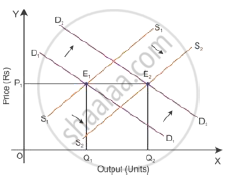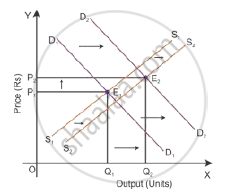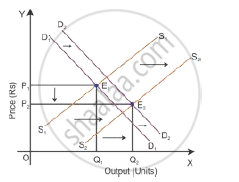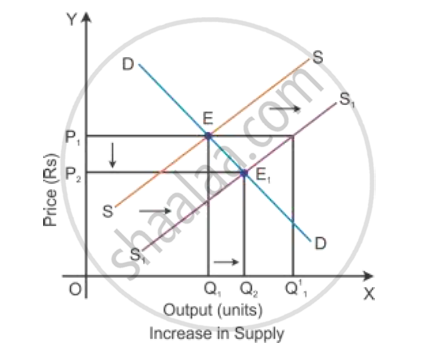# Market for a Good is in Equilibrium. There is Simultaneous "Increase" Both in Demand and Supply of the Good. Explain Its Effect on Market Price - Economics

A market for a good is in equilibrium. There is simultaneous "increase" both in demand and supply of the good. Explain its effect on the market price

Market for a good is in equilibrium. Supply of the good 'increases'. Explain the chain of effects of this change.

#### Solution 1

When there is a simultaneous increase in demand and supply, the equilibrium point of price of output will depend on the basis of proportion of changes in demand and supply.

Let us understand the effects through the following conditions:
i. If increase in demand is equal to increase in supply
When the demand increases from D1D1 to D2D2 and the supply increases from S1S1 to S2S2 in the same proportion, the new equilibrium price will be OP1 and the new equilibrium output will be OQ2. This new price and output intersect each other at Point E2 and will arrive at new equilibrium. As the increase in demand is equal to the increase in supply, the price remains the same at the new equilibrium point.ii. If increase in demand is more than increase in supply
When the demand increases, the demand curve will shift to D2D2 and the increase in supply will shift the supply curve to S2S2. Here, the increase in demand is more than the supply; hence, there will be a new equilibrium price and a new equilibrium output which intersects at Point E2. As the increase in demand is more than the decrease in supply, the equilibrium price increases at the new equilibrium point.iii. If increase in demand is less than increase in supply
When the demand increases, the demand curve will shift to D2D

When there is a simultaneous increase in demand and supply, the equilibrium point of price of output will depend on the basis of proportion of changes in demand and supply.

Let us understand the effects through the following conditions:
i. If increase in demand is equal to increase in supply
When the demand increases from D1D1 to D2D2 and the supply increases from S1S1 to S2S2 in the same proportion, the new equilibrium price will be OP1 and the new equilibrium output will be OQ2. This new price and output intersect each other at Point E2 and will arrive at new equilibrium. As the increase in demand is equal to the increase in supply, the price remains the same at the new equilibrium point.ii. If increase in demand is more than increase in supply
When the demand increases, the demand curve will shift to D2D2 and the increase in supply will shift the supply curve to S2S2. Here, the increase in demand is more than the supply; hence, there will be a new equilibrium price and a new equilibrium output which intersects at Point E2. As the increase in demand is more than the decrease in supply, the equilibrium price increases at the new equilibrium point.iii. If increase in demand is less than increase in supply
When the demand increases, the demand curve will shift to D2D2 and the increase in supply will shift the supply curve to S2S2. Here, the increase in demand is less than the supply; hence, there will be a new equilibrium price and a new equilibrium output which intersects at Point E2. As the increase in demand is less than the increase in supply, the equilibrium price decreases at the new equilibrium point.#### Solution 2

Consider DD to be the initial demand curve and SS to be the supply curve of the market. Market equilibrium is achieved at Point E, where the demand and supply curves intersect each other. Therefore, the equilibrium price is OP, and the equilibrium quantity demanded is OQ.

When there is the change in other factors than price, there will be the rise in the supply of goods. There will be a shift in the supply curve towards the right to SS1 with an increase in the supply, and the demand curve DD will remain the same. This implies that there will be a situation of excess supply at the equilibrium point.In the above diagram, there is an excess supply of OQ1 to OQ11 units of output at the initial price OP1. Thereby the producers will tend to reduce the price of the output to increase the sale in the market. A profit margin of the firm will come down and slowly some of the firms will tend to quit the market. Because of this, the market supply will decline to OQ2 level of output and the price of the output also gets reduced to the point OP2. Now, the new market equilibrium will be at Point E1, where the new supply curve SS1 intersects the demand curve DD.

Concept: Market Equilibrium
Is there an error in this question or solution?
2011-2012 (March) Delhi Set 1
Share